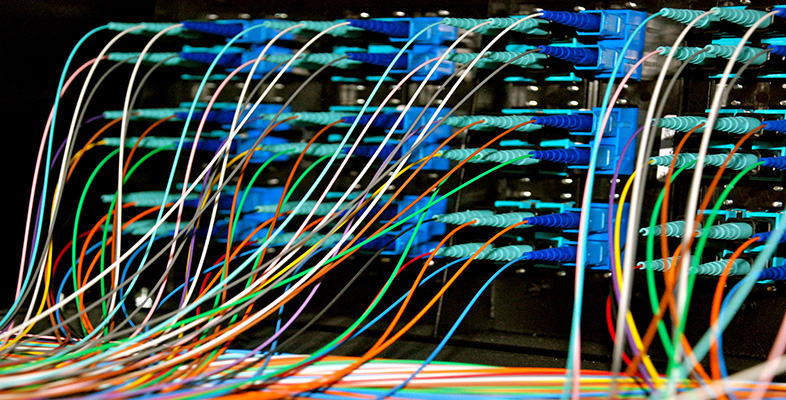Representing and manipulating data in computers

Start this free course now. Just create an account and sign in. Enrol and complete the course for a free statement of participation or digital badge if available.

Free course

# 5 Representing data in computers: conclusion

Study note: You will need to refer to the Reference Manual while you are working through this section.

View document [Tip: hold Ctrl and click a link to open it in a new tab. (Hide tip)]

There is one very important type of data that I need to introduce before I leave this topic of representing data in computers. This type of data didn't fit neatly into just one of Sections 2, 3 or 4 because it is a type found in all computers. This data type relates to the computer programs that enable the processor to carry out tasks. A computer program that is ready to be executed on a processor will be in the form of a long list of computer instructions, and you will not be surprised to learn that each computer instruction is encoded as one or more binary words.

Every make and model of processor is designed to have a particular set of instructions which it can execute, and one of the tasks of the processor's designers is to decide which binary code will represent each of these instructions.

Most programmers, however, do not need to be aware of the binary codes that represent the processor's instructions when they write a program. This is because there are computer programs called ‘compilers’ which take a program written in a form easy for humans to work in and translate it into the binary codes the processor needs.

The last few sections have demonstrated that every conceivable type of data is encoded in computers in binary form. Did it perhaps occur to you that this means that codes – patterns of 1s and 0s – can stand for different entities in different situations? Take for example the code

0100 0001

If this code word represents text then it stands for the letter ‘A’ in the 8-bit ASCII coding scheme. If it represents an integer then it stands for 65 in the natural binary coding scheme. If it represents a set of true/false quantities then it could represent, say, which segments of a seven-segment display were on or off. If it is part of the representation of an image then it will encode a particular level of brightness and/or colour. If it is part of the representation of a computer program then it could represent, say, an instruction to perform an addition.

How does the computer ‘know’ how to treat a particular code?

The answer to this is that it does exactly what the program tells it to do with each code it meets. The very first code a processor encounters when it is first switched on must be the code for a computer instruction. This will tell the processor what to do next, and so on. From then on the computer will do whatever manipulations it is told to do on every binary code word it is given. It is up to the people who write the program or programs that run on the computer to ensure that the computer is given the right code words, in the right order, and is correctly told what to do with each and every one of them.

This is why software is such a crucial part of a computer system.

## Activity 22 (Self assessment)

What would the code word

0010 0100

stand for if it is:

1. the code for an integer using natural binary;

2. the code for a fixed-point fraction where the binary point is taken to lie between the two groups of 4 bits;

3. the code for a set of 8 Boolean variables which represent whether the segments of a 7-segment display are on or off (assume the same convention as given in Section 2.6);

4. the code for a text character using the same 8-bit ASCII code as in a PC?

1. In natural binary, this represents 32 + 4 = 36.

2. If the binary point lies between the two groups of 4 bits, then the integer part of the fraction is 0010, which is 2, and the fractional part is 0100, which is ¼. Hence the fraction is 2¼.

3. Segments 5 and 2 are on, the others are off. Reference to Figure 5 shows that the digit 1 will be displayed.

4. The table in the appendix of the Reference Manual shows that this code represents the ‘\$’ symbol.